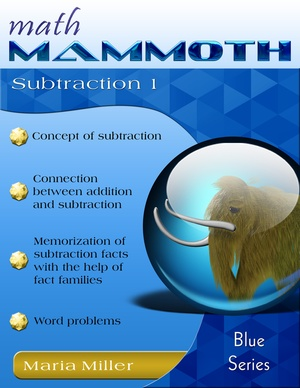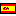^
You are here: HomeBlue Series → Subtraction 1

# Math Mammoth Subtraction 189 pages

## Prices & ordering

Printed copy \$12.50

(credit cards, Google Pay, Paypal, PO)

Printed copy £5.64
US shipping availableEste libro en español

Math Mammoth Subtraction 1 deals with various concepts related to basic subtraction, and with basic addition and subtraction facts within 0-10. Most of the problems in the book only use numbers up to 10, but a few include numbers between 10 and 20.

The PDF version of this book is enabled for annotation. This means that if you prefer, the student can fill it in on the computer, using the typewriter and drawing tools in Adobe Reader version 9 or greater.

The concept of subtraction is easy to illustrate with the idea of "taking away". If your child does not yet know the word "minus", it is a good idea to introduce it first orally. Simply use blocks, rocks, or other concrete objects. For example, show the child eight blocks, and take away three blocks. Then use both kinds of wordings: "Eight blocks, take away three blocks, leaves five blocks. Eight blocks minus three blocks equals five blocks."

Play with the blocks or other concrete objects until the child can use the words "minus" and "equals" in his/her own speech. This will make it much easier to introduce the actual written symbols.

The next step would be to abandon concrete objects and use semi-concrete illustrations or pictures. That is where this book starts with the lesson Subtraction Is "Taking Away". At this stage, the child can still figure out the subtraction problems by simply counting how many objects are left.

So, how does the student learn how to subtract without actually counting concrete objects or pictures? As a transitional strategy, we will study counting down: the student solves 9 − 3, for example, by counting down three steps from nine: eight, seven, six. So the answer is six.

However, the final goal is to learn to use the addition facts to find the answer to subtraction problems. For example, once the student knows that 5 + 5 = 10, then this fact is used to solve 10 − 5 = 5. For this purpose, the student must learn well the connection between addition and subtraction. This is why this book concentrates heavily on the connection between addition and subtraction with several lessons, ending up with the concept of fact families.

Besides "taking away", subtraction is also used for these two situations:

• Finding how much more one number is than another. Note that no one "takes away" anything in this situation. For example, if you have 3 dollars and you need 6 dollars, how many more dollars do you need? The student is instructed to write a "how many more" addition problem for this, which looks like this: 3 + ___ = 6. We also call these problems "missing addend" problems. It can be solved by remembering the addition fact 3 + 3 = 6, or by subtracting 6 − 3 = 3.
• Two (or more) parts (of something) make up a whole. If you know the whole and one of its parts, you can figure out the other part. For example, if there are 10 white and red flowers, and seven of them are white, how many are red? We know the "parts" (the red and white flowers) add up to 10, so we write an addition 7 + __ = 10. Again, this can be solved by subtracting, or simply by knowing the addition fact 7 + 3 = 10.

These two situations are dealt with in several lessons in the book and are found in various word problems throughout the book.

In the latter part of the book, we encounter several lessons named Addition and Subtraction Facts with... They aim at helping the child to memorize the basic addition and subtraction facts. We are approaching it from the concept of fact families.

These lessons have a lot of practice problems. Use your judgment as to whether your child will need to do all of the problems. If he/she masters the facts quickly, you can skip some of them. 4

Besides the written problems, I encourage you to use games that are explained below. Children like to play, and using the addition and subtraction facts in a game gives them fun and education in the same "package".

While this book is not tied to any grade level, it is most suitable for first grade.

You might also be interested in:
• Math Mammoth Addition 1—a worktext about addition concept within 0-10, sum families (sums with 7, 8, etc.), and word problems (grade 1).
• Math Mammoth Add & Subtract 2-A—a worktext about "easy" additions and subtractions within 0-100, memorizing the basic addition facts of single-digit numbers, and word problems (grades 1-2).
• Math Mammoth Place Value 1—a worktext about two-digit numbers (place value), comparing, rounding, even & odd, and graphs (grade 1).
• Free subtraction worksheets for grade 1 — includes single-digit subtraction, double-digit subtraction, subtracting multiples of ten, and missing number subtraction problem worksheets.

## Customer Testimonials

Maria,

My 4 year old just finished Subtraction 1. We've done Addition 1 and Place Value 1 this fall as well. He loves it! I found myself frustrated with Saxon Math K and found Math Mammoth last summer. We're going to breeze through Light Blue 1 this spring and have fun committing his math facts to memory. Thank you for a simple straightforward math program!

Amanda Pelser
http://thepelsers.com

## Better Yet - Bundle Deals!

*BONUS*: Buy any bundle listed above, and get the fully upgraded Soft-Pak software for FREE (includes 4 math and 2 language arts programs, complete with on screen and printable options).

## How and where to order

You can buy Math Mammoth books at:

• Here at MathMammoth.com website — simply use the "Add to cart" buttons you see on the product pages.
• Rainbow Resource carries printed copies for the Light Blue series books, plus several CDs (Light Blue and Blue series).
• At Teachers Pay Teachers you can purchase the Light Blue Series downloads, plus topical units.
• Lulu sells printed copies for most of the Math Mammoth materials (various series).

By purchasing any of the books, permission IS granted for the teacher (or parent) to reproduce this material to be used with his/her students in a teaching situation; not for commercial resale. However, you are not permitted to share the material with another teacher.

In other words, you are permitted to make copies for the students/children you are teaching, but not for other teachers' usage.

Math Mammoth books are PDF files. You will need Adobe Reader to view them, including if you use a Mac or Linux. You can try other PDF viewers, but they seem to either omit or mess up some of the images.
WAIT!

Receive my monthly collection of math tips & resources directly in your inbox — and get a FREE Math Mammoth book!You can unsubscribe at any time.

### Math Mammoth Tour

Confused about the different options? Take a virtual email tour around Math Mammoth! You'll receive:

An initial email to download your GIFT of over 400 free worksheets and sample pages from my books. Six other "TOURSTOP" emails that explain the important things and commonly asked questions concerning Math Mammoth curriculum. (Find out the differences between all these different-colored series!)

This way, you'll have time to digest the information over one or two weeks, plus an opportunity to ask me personally about the curriculum.
A monthly collection of math teaching tips & Math Mammoth updates (unsubscribe any time)### "Mini" Math Teaching Course

This is a little "virtual" 2-week course, where you will receive emails on important topics on teaching math, including:

- How to help a student who is behind
- Troubles with word problems
- Teaching multiplication tables
- Why fractions are so difficult
- The value of mistakes
- Should you use timed tests
- And more!

A GIFT of over 400 free worksheets and sample pages from my books right in the very beginning.A monthly collection of math teaching tips & Math Mammoth updates (unsubscribe any time)
Enter your email to receive math teaching tips, resources, Math Mammoth news & sales, humor, and more! I tend to send out these tips about once monthly, near the beginning of the month, but occasionally you may hear from me twice per month (and sometimes less often).• A GIFT of over 400 free worksheets and sample pages from my books.